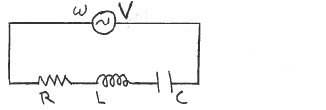# Problem: In an R-L-C ac series circuit the source voltage amplitude is 360 V and its angular frequency ω is equal to the resonance frequency of the circuit. R = 90.0 Ω, C = 8.00 x 10-6 F, and L = 2.0 H. What is the voltage amplitude VL for the inductor?

###### Problem Details

In an R-L-C ac series circuit the source voltage amplitude is 360 V and its angular frequency ω is equal to the resonance frequency of the circuit. R = 90.0 Ω, C = 8.00 x 10-6 F, and L = 2.0 H.

What is the voltage amplitude VL for the inductor?